You are currently offline. Some features of the site may not work correctly.

# Rigidity matroid

In the mathematics of structural rigidity, a rigidity matroid is a matroid that describes the number of degrees of freedom of an undirected graph… Expand
Wikipedia

## Papers overview

Semantic Scholar uses AI to extract papers important to this topic.
2013
2013
• Eur. J. Comb.
• 2013
• Corpus ID: 13816612
Let M be a d-dimensional generic rigidity matroid of some graph G. We consider the following problem, posed by Brigitte and… Expand
•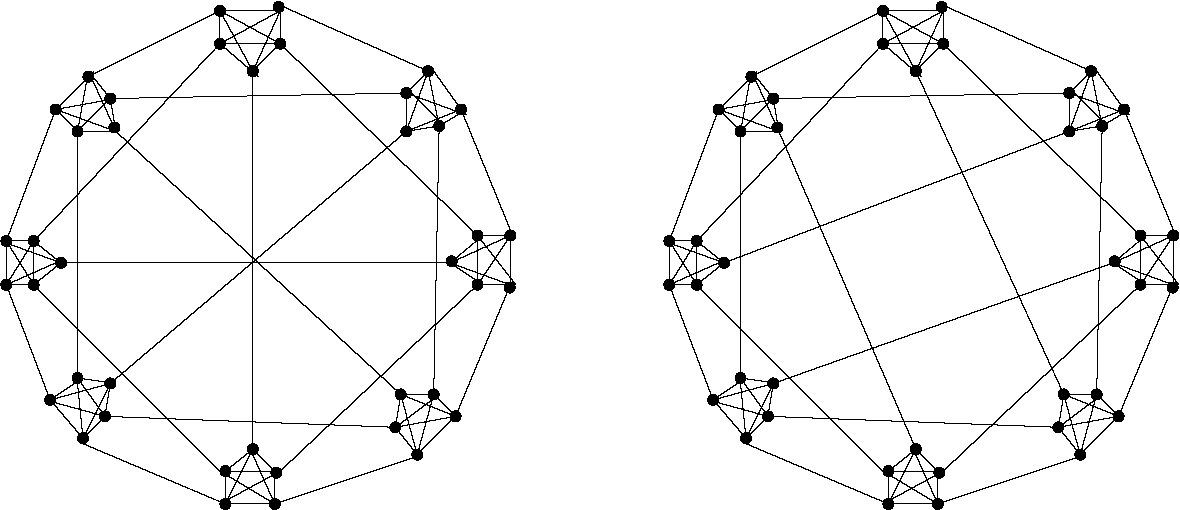•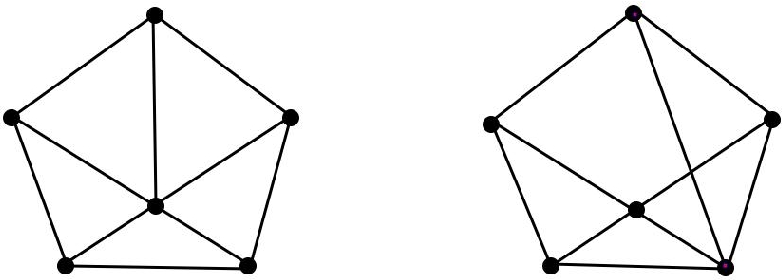Is this relevant?
2010
2010
The problem of characterizing the generic rigidity matroid combinatorially is completely solved in dimension 2 but still open in… Expand
•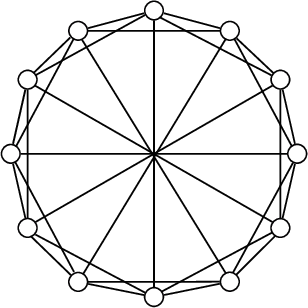•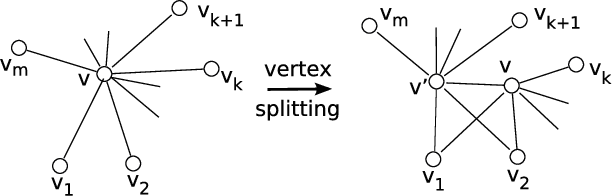•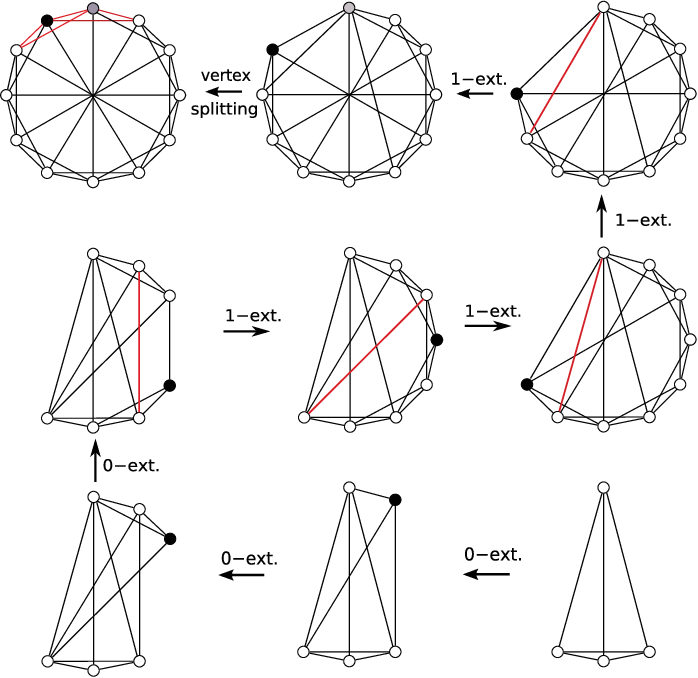•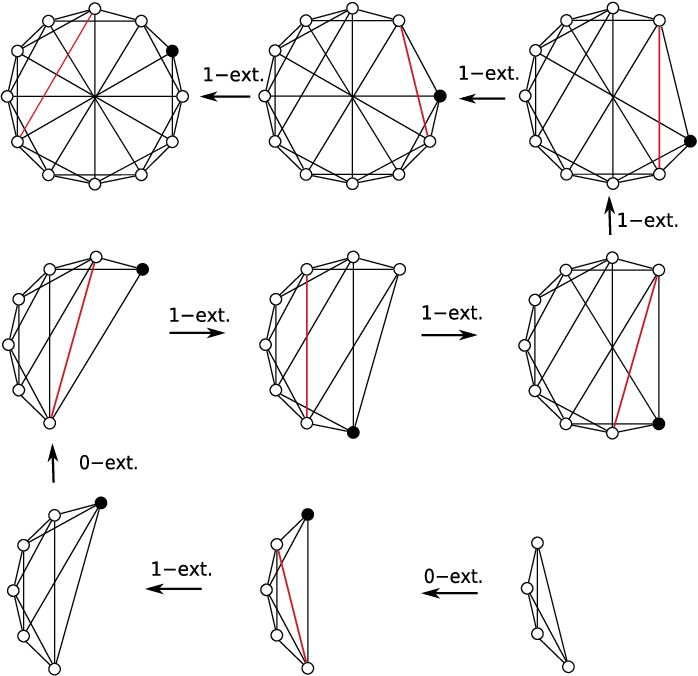Is this relevant?
2010
2010
• J. Comb. Theory, Ser. B
• 2010
• Corpus ID: 34218632
A two-dimensional mixed framework is a pair (G,p), where G=(V;D,L) is a graph whose edges are labeled as 'direction' or 'length… Expand
Is this relevant?
2008
2008
Let H=(V,E) be a hypergraph and let k≥1 and l≥0 be fixed integers. Let M be the matroid with ground-set E s.t. a set F⊆E is… Expand
Is this relevant?
2006
2006
In this paper we consider the 3-dimensional rigidity matroid of squares of graphs. These graphs are also called molecular graphs… Expand
•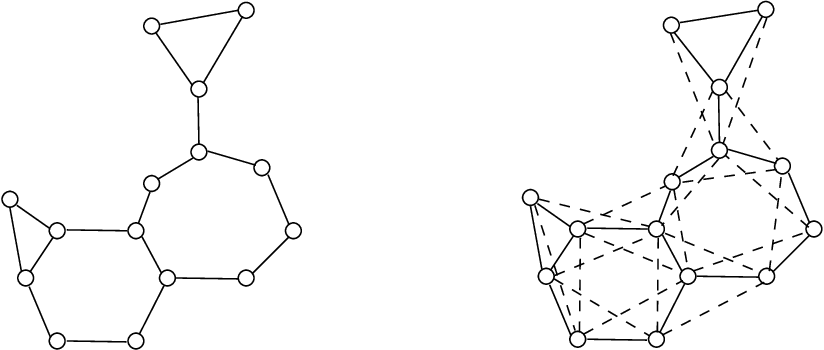•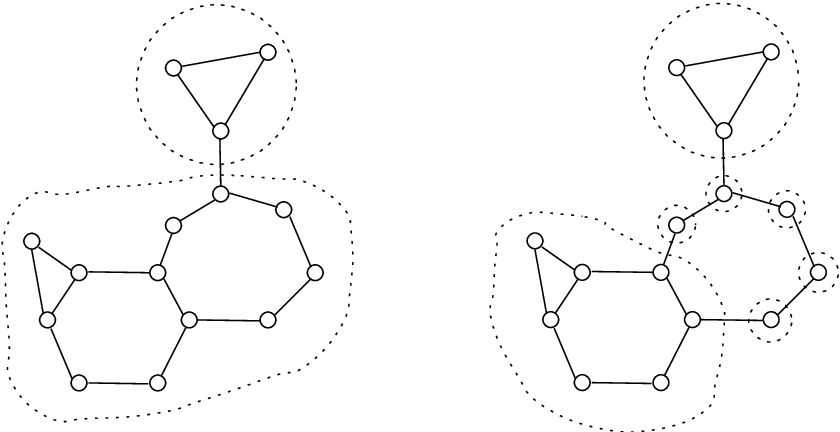Is this relevant?
Highly Cited
2005
Highly Cited
2005
• J. Comb. Theory, Ser. B
• 2005
• Corpus ID: 18561398
A d-dimensional framework is a straight line realization of a graph G in Rd. We shall only consider generic frameworks, in which… Expand
•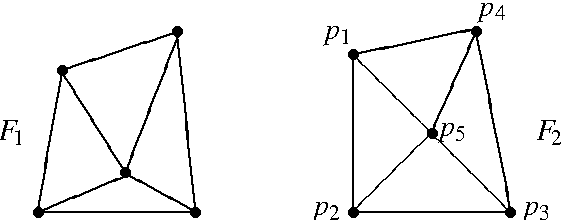•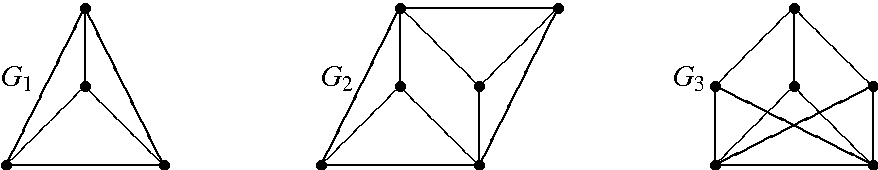•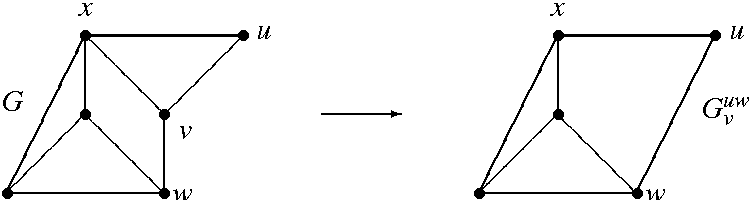•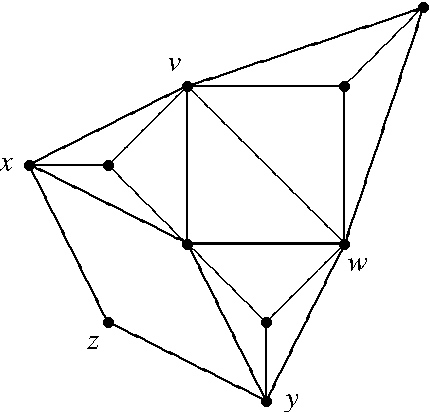•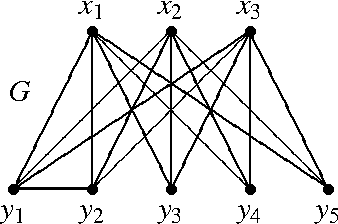Is this relevant?
2005
2005
• J. Comb. Theory, Ser. B
• 2005
• Corpus ID: 13850416
Let Rd(G) be the d-dimensional rigidity matroid for a graph G = (V, E). For X ⊆ V let i(X) be the number of edges in the subgraph… Expand
Is this relevant?
2004
2004
Let ${\mathcal R}_{d}(G)$ be the d-dimensional rigidity matroid for a graph G=(V,E). Combinatorial characterization of… Expand
Is this relevant?
Highly Cited
2003
Highly Cited
2003
• J. Comb. Theory, Ser. B
• 2003
• Corpus ID: 43350566
A graph G = (V , E) is called a generic circuit if |E| = 2|V| - 2 and every X ⊂ V with 2 ≥ |X| ≥ |V| - 1 satisfies i(X) ≤ 2|X… Expand
Is this relevant?
1991
1991
Abstract We give a characterization of the dual of the 2-dimensional generic rigidity matroid R ( G ) of a graph G and derive… Expand
Is this relevant?